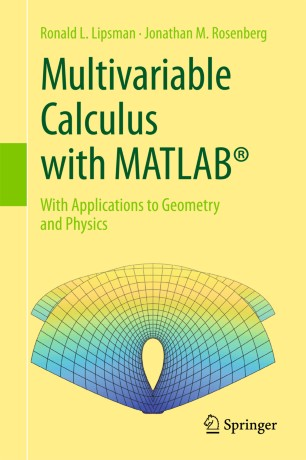Multivariable Calculus with MATLAB®

With Applications to Geometry and Physics

• Ronald L. Lipsman
• Jonathan M. RosenbergTextbook

1. Front Matter
Pages i-xii
2. Ronald L. Lipsman, Jonathan M. Rosenberg
Pages 1-13
3. Ronald L. Lipsman, Jonathan M. Rosenberg
Pages 15-31
4. Ronald L. Lipsman, Jonathan M. Rosenberg
Pages 33-60
5. Ronald L. Lipsman, Jonathan M. Rosenberg
Pages 61-73
6. Ronald L. Lipsman, Jonathan M. Rosenberg
Pages 75-94
7. Ronald L. Lipsman, Jonathan M. Rosenberg
Pages 95-121
8. Ronald L. Lipsman, Jonathan M. Rosenberg
Pages 123-146
9. Ronald L. Lipsman, Jonathan M. Rosenberg
Pages 147-183
10. Ronald L. Lipsman, Jonathan M. Rosenberg
Pages 185-203
11. Ronald L. Lipsman, Jonathan M. Rosenberg
Pages 205-234
12. Ronald L. Lipsman, Jonathan M. Rosenberg
Pages 235-240
13. Ronald L. Lipsman, Jonathan M. Rosenberg
Pages 241-267
14. Back Matter
Pages 269-276

Introduction

This comprehensive treatment of multivariable calculus focuses on the numerous tools that MATLAB® brings to the subject, as it presents introductions to geometry, mathematical physics, and kinematics. Covering simple calculations with MATLAB®, relevant plots, integration, and optimization, the numerous problem sets encourage practice with newly learned skills that cultivate the reader’s understanding of the material. Significant examples illustrate each topic, and fundamental physical applications such as Kepler’s Law, electromagnetism, fluid flow, and energy estimation are brought to prominent position. Perfect for use as a supplement to any standard multivariable calculus text, a “mathematical methods in physics or engineering” class, for independent study, or even as the class text in an “honors” multivariable calculus course, this textbook will appeal to mathematics, engineering, and physical science students.

MATLAB® is tightly integrated into every portion of this book, and its graphical capabilities are used to present vibrant pictures of curves and surfaces. Readers benefit from the deep connections made between mathematics and science while learning more about the intrinsic geometry of curves and surfaces. With serious yet elementary explanation of various numerical algorithms, this textbook enlivens the teaching of multivariable calculus and mathematical methods courses for scientists and engineers.

Keywords

Multivariable Calculus Geometry of Curves Vectors and Graphs Kinematics Directional derivatives MatLab vectors geometry of surfaces optimization multiple integrals multidimensional calculus physical applications of vector calculus vector calculus

Authors and affiliations

• Ronald L. Lipsman
• 1
• Jonathan M. Rosenberg
• 2
1. 1.Department of Mathematics University of MarylandROCKVILLEUSA
2. 2.William E Kirwan HallUniversity of Maryland, Mathematics Dept William E Kirwan HallCollege ParkUSA

Bibliographic information

Industry Sectors
Electronics